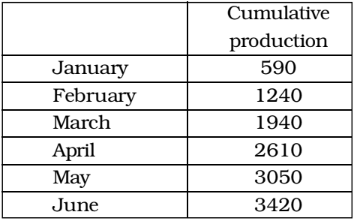## Introduction to Data Interpretation

#### Data Interpretation

1. Refer the following data table and answer the following question.If 400 calories are burnt by jogging 5 km, how many calories were burnt in the given week?

1. As per the given above table , we see
Total distance covered = (3 + 2 + 2.5 + 5 + 1 + 2.5 + 4) km. = 20 km.
∵ 5 km. ≡ 400 calories

##### Correct Option: D

As per the given above table , we see
Total distance covered = (3 + 2 + 2.5 + 5 + 1 + 2.5 + 4) km. = 20 km.
∵ 5 km. ≡ 400 calories

 ∴ 20 km. ≡400 × 100calories 5

≡ 1600 calories

1. Refer the following data table and answer the following question.How many cars were manufactured in the months of April and May?

1. On the basis of given table in question ,
Respective production :
January ⇒ 590
February ⇒ 1240 – 590 = 650
March ⇒ 1940 – 1240 = 700
April ⇒ 2610 – 1940 = 670
May ⇒ 3050 – 2610 = 440

##### Correct Option: D

On the basis of given table in question ,
Respective production :
January ⇒ 590
February ⇒ 1240 – 590 = 650
March ⇒ 1940 – 1240 = 700
April ⇒ 2610 – 1940 = 670
May ⇒ 3050 – 2610 = 440
∴ Total production of cars in April and May = 670 + 440 = 1110

Direction: Study the table and answer the questions. The number of 5 types of cycles manufactured by a company over the years is given below :1. The production of E type of cycle in 2001 was what percent of production of B type in 2000?

1. As per the given above table , we see
The production of E type of cycle in 2001 = 80 thousands
The production of B type of cycle in 2000 = 160 thousands

 Required percent = The production of E type of cycle in 2001 × 100 % The production of B type of cycle in 2000

##### Correct Option: B

As per the given above table , we see
The production of E type of cycle in 2001 = 80 thousands
The production of B type of cycle in 2000 = 160 thousands

 Required percent = The production of E type of cycle in 2001 × 100 % The production of B type of cycle in 2000

 Required percent = 80 × 100 = 50 % 160

1. What was the percentage drop in production of A-type cycle from 1997 to 1999?

1. According to given table, we have
Production of A-type cycle in 1997 = 200
Production of A-type cycle in 1999 = 180
Decrease = 200 - 180 = 20

 Required percentage decrease = Decrease × 100 Production of A-type cycle in 1997

##### Correct Option: A

According to given table, we have
Production of A-type cycle in 1997 = 200
Production of A-type cycle in 1999 = 180
Decrease = 200 - 180 = 20

 Required percentage decrease = Decrease × 100 Production of A-type cycle in 1997

 Required percentage decrease = 20 = 10 % 2

1. In the case of which type of cycles was total production of the given 5 years the maximum?

1. On the basis of given table in question ,
Total production of cycles :
Type–A ⇒ 945
Type–B ⇒ 850
Type–C ⇒ 520
Type–D ⇒ 565

##### Correct Option: A

On the basis of given table in question ,
Total production of cycles :
Type–A ⇒ 945
Type–B ⇒ 850
Type–C ⇒ 520
Type–D ⇒ 565
Type–E ⇒ 375
Therefore , A type of cycle is required answer .